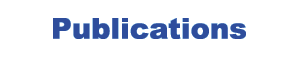# Abstract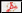= PDF Reprint,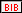= BibTeX entry,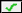= Online Abstract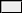L. Itti, C. Koch, J. Braun, A Model for the Attentional Modulation of Spatial Vision, In: Investigative Ophthalmology and Visual Science (Proc. ARVO 1997), Vol. 38, No. 4, p. 5461, Mar 1997. (Cited by 3)

Abstract: Purpose: We present a computational model of interactions between visual spatial filters in humans. The model accounts for a range of visual spatial thresholds and their modulation by attention (Wen et al., ARVO'97). Methods: The model assumes that each visual location is analyzed by a set of spatial filters tuned for different spatial frequencies \$(lambda)\$ and orientations \$(theta)\$. Pairs of filters in quadrature phase produce an energy response, \$E_{lambda,theta}\$, which is normalized by the responses \$E_{lambda',theta'}\$ of a subset of filters, centered around \$(lambda,theta)\$ and weighted by coefficients \$w_{lambda,theta}(lambda',theta')\$, yielding the pooled energy: \$\${cal E}_{lambda,theta} = {{E_{lambda,theta}^{gamma}}over {sigma_{lambda}^{delta}+sum_{lambda',theta'} w_{lambda,theta}(lambda',theta') E_{lambda',theta'}^{delta}}} qquad (gamma approx 2; delta approx 1.5, without attention)\$\$ Psychophysical performance is derived by assuming that each filter exhibits constant background noise plus Poisson noise and by selecting the most sensitive filter for each task (ROC analysis). Results: The model is similar to models of contrast gain control in cat simple cells (Heeger, Vis. Neurosci. 9:181-97, 1992) and produces, without additional assumptions, the ``dip-shaped'' non-linearity characteristic of human threshold vision (Wilson et al., Vis. Res. 23:873--82, 1983). Our model differs from others in that only a relatively narrow normalization pool (halfwidth of weight function \$approx 1octave\$ and \$approx 15^circ\$) accounts for human vision. The model successfully reproduces human contrast, contrast increment, and orientation discrimination thresholds, as well as threshold elevation by contrast masking (Wen et al., ARVO'96 and '97). Increasing both exponents \$gamma\$ and \$delta\$ accounts for the effect of attention. Conclusion: The results suggest that attention increases the gain and sharpens the tuning of visual spatial filters, by strengthening normalizing interactions between such filters.

Copyright © 2000-2007 by the University of Southern California, iLab and Prof. Laurent Itti.
This page generated by bibTOhtml on Tue 10 Jan 2023 02:30:30 PM PST